In the previous post, I mentioned I was starting to read Parallel and Concurrent Programming in Haskell, but that was motivated by wanting to shorten render times as I worked through Ray Tracing in One Weekend by dividing the work across the two cores of my aging laptop.

The below image would take about 17 minutes to render on a single core (after trying lots of compiler optimizations), and about 11 minutes to render after making a few edits to use parMap rpar from Chapter 2 of Parallel and Concurrent Programming. (Hooray for quick wins!)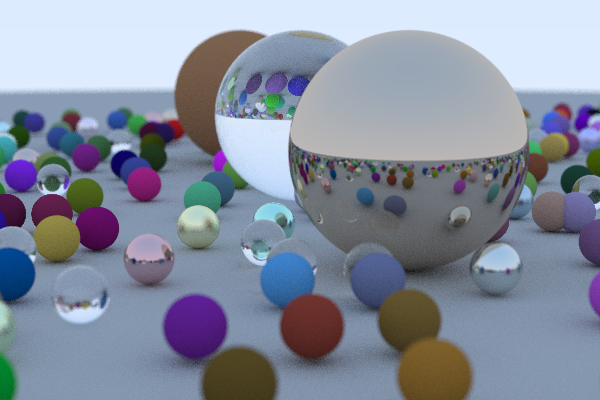## The problem

Unfortunately, the scene is so busy that it masked a bug in my implementation that caused fine lines to appear on the surfaces of objects, like a brushed metal effect:This effect is also visible with two constant colored Lambertian spheres (that don't look so "constant" due to this bug):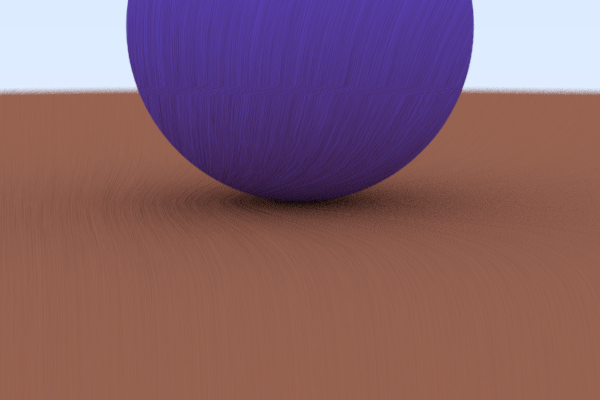I kept thinking it might have been some divide-by-zero singularity, because the lines seem to follow the curves of some of the shapes in the image. For example, the bottom of the small sphere is tangent to the lower sphere at point (0, 0, 0), and the lines at the bottom seem to curve around that origin spot. However, while digging through the code revealed a few other minor errors, nothing stood out as being directly related to a singularity at the global origin.

Rendering the random scene from above revealed that my earlier tests on the parallel renderer might have had similar problems: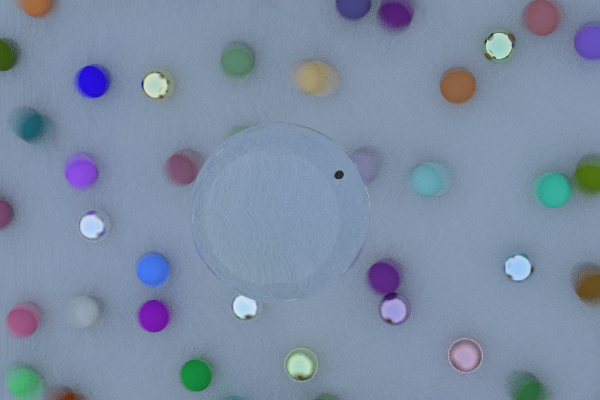I can see similar lines in the gray ground, where the lines bend around the various small spheres. The lines are also visible inside the large dielectric sphere in the middle of this image.

## Where did things go wrong?

One thing I noticed is that the lines generally seemed to extend "vertically" in the image, but also seemed to interact with the shapes.

This gave me a hunch that my problem was in random number generation, or more particularly, my mismanagement of the random number generator when parallelizing the code.

This ray tracer uses randomness to generate smoother images in a variety of ways. These include randomizing a direction of reflection of rays that bounce off Lambertian (e.g., diffuse or matte) surfaces or reflections off metal surfaces that have some "fuzziness" (e.g., matte qualities).

Randomness is also used to anti-alias the generated image by computing multiple color samples for each pixel. For example, the basic ray tracer from the beginning of the book generated one sample for each pixel by tracing a ray that passed through the center of the pixel. However, this results in aliasing effects at the edges of objects, as the sample rate is much lower than the Nyquist rate of the scene. To address this, multiple samples (e.g., $$total samples = 100$$ samples) are taken for each pixel by passing rays through random locations within the 2-D area of the pixel, and the resulting output color of the rendering operation is the average color of all these samples.

Here's the main outer "loop":

render :: IO ()
render = do
putStrLn "P3"
putStrLn $show imageWidth ++ " " ++ show imageHeight putStrLn "255" let pp = pixelPositions imageWidth imageHeight let gen = pureMT 1024 -- Fix a seed for comparable performance tests let (world, g1) = makeTwoPerlinSpheresScene 0.0 1.0 gen let camera = randomSceneCamera gs <- replicateM (nThreads - 1) newPureMT let gens = g1 : gs let vals = runST$
mapM
(\rowPs ->
map scaleColors <$> parallelRenderRow rowPs world camera gens) pp mapM_ printRow (zip [1 .. imageHeight] vals) hPutStr stderr "\nDone.\n"  The key parts of the above code are the definition of vals and the line mapM_ printRow (zip [1 .. imageHeight] vals). vals defines the output colors of the rows of pixels and the mapM line prints those out, row-by-row to stdout in PPM format. By using the lazy version of the ST monad (Control.Monad.ST.Lazy), each row is only evaluated when it is needed to print the row. As part of the definition of vals, I run an ST monad that essentially maps a parallelRenderRow function over pp :: [[(Int, Int)]], which is a list of lists of pixel coordinates. parallelRenderRow takes a given row of pixel coordinates and calculates color values ("Albedo") for each of those pixels. The map scaleColors converts those from Albedo values from Doubles in the range (0, 1) to Int values in the range (0, 255). To run this in parallel over nThreads, I create a separate random number generator for each of the nThreads using newPureMT (I'm using the mersenne-random-pure64 library, which is much faster than the bundled System.Random functions). These nThreads random number generators are put into a list gens, which is passed in to parallelRenderRow. parallelRenderRow :: [(Int, Int)] -> Scene -> Camera -> [PureMT] -> ST s [Albedo] parallelRenderRow rowps world camera gs = let sampleGroups = parMap rpar (\gen -> force$ runST $do worldRef <- newSTRef world genRef <- newSTRef gen runReaderT (renderRow rowps) (worldRef, camera, genRef)) gs in return$ foldl'
(zipWith ($$Albedo a1) (Albedo a2) -> Albedo  vecAdd a1 a2)) (replicate imageWidth (Albedo  Vec3 (0.0, 0.0, 0.0))) sampleGroups  Here, in parallelRenderRow, the idea is to use \(n$$ different threads to compute pixel colors for their own $$total samples / n$$ samples render the same row and then averaging the colors computed by the $$n$$ threads. However, these $$n$$ threads need to use $$n$$ independent random number generators. If they all used the same random number generator, then they would compute identical outputs, and therefore there would be no visual benefit.

For example, in the main render code above, I generated one of the random generators using a fixed seed of 1024: let gen = pureMT 1024. I generated the additional generators using newPureMT, which generates a seed based on the current time. This particular library allows you to generate, for example, Double values using the randomDouble :: PureMT -> (Double, PureMT by passing in a PureMT. The output is the requested Double and new PureMT for use the next time we need a random number. Reusing the original PureMT would only give us the same random number generated earlier.

λ> g1 <- newPureMT                -- Create a first PureMT
λ> (rd1, g2) = randomDouble g1    -- Generate a double and another PureMT
λ> rd1
0.15164889407071558               -- value of generated double rd1
λ> g2
"<PureMT>"                        -- "value" of generated PureMT g2
λ> (rd2, g3) = randomDouble g2    -- Generate another double and PureMT
λ> rd2
0.2577711144917828                -- value of generated double rd2
λ> randomDouble g1                -- calling randomDouble with the original
(0.15164889407071558,"<PureMT>")  -- PureMT generates the same rd1 value
λ> randomDouble g2                -- calling randomDouble with the second PureMT
(0.2577711144917828,"<PureMT>")   -- generates the same rd2 value


So I use parMap with strategy rpar over the generators gs to run an ST monad on the supplied Scene and with the generator gen for the given thread. This gives a list of sampleGroups, and we foldl' these up using vecAdd, which adds corresponding components of the color vectors.

As it turns out, when rendering each row, I used the same random number generators in the same states, rather than passing along the random number generator state from the previous row. In particular, in the main render function, the same generators gens are supplied when rendering each row. I knew what I was trying to do, because I used mapM to try to thread the random number generators through the computations, but messed that one up.

## The fix

The fix was to make sure that the proper new random number generators, in their states after rendering a given row, were fed into the call to parallelRenderRow for each next row. mapM can handle that for us, so long as the updated PureMTs are stored in the ST monad.

render :: IO ()
render = do
putStrLn "P3"
putStrLn $show imageWidth ++ " " ++ show imageHeight putStrLn "255" let pp = pixelPositions imageWidth imageHeight let gen = pureMT 1024 -- Fix a seed for comparable performance tests let (world, g1) = makeTwoPerlinSpheresScene 0.0 1.0 gen let camera = randomSceneCamera gs <- replicateM (nThreads - 1) newPureMT let gens = g1 : gs let vals = runST$ do
gensRef <- newSTRef gens
mapM
(\rowPs ->
map scaleColors <$> parallelRenderRow rowPs world camera gensRef) pp mapM_ printRow (zip [1 .. imageHeight] vals) hPutStr stderr "\nDone.\n"  The main change here war to create a create a gensRef that was an STRef to a list of PureMTs and passing that STRef s [PureMT] to parallelRenderRow. parallelRenderRow :: [(Int, Int)] -> Scene -> Camera -> STRef s [PureMT] -> ST s [Albedo] parallelRenderRow rowps world camera gsRef = do gs <- readSTRef gsRef let (sampleGroups, newGs) = unzip$
parMap
rpar
(\gen ->
force $runST$ do
worldRef <- newSTRef world
genRef <- newSTRef gen
(do row <- renderRow rowps
g <- lift $readSTRef genRef return (row, g)) (worldRef, camera, genRef)) gs writeSTRef gsRef newGs return$
foldl'
(zipWith (\(Albedo a1) (Albedo a2) -> Albedo $vecAdd a1 a2)) (replicate imageWidth (Albedo$ Vec3 (0.0, 0.0, 0.0)))
sampleGroups


The changes here were to update the type signature to change [PureMT] to STRef s [PureMT], and then read the [PureMT] from the ST monad.

To get the final PureMT random number generators back from each thread, I had to g <- lift \$ readSTRef genRef, so that each thread would return its computed colors row and its final random generator g, which meant that the output of the parMap had type [([Albedo], PureMT)]. Using unzip lets us extract the sampleGroups and the newGs. We can then write the newGs back into the ST monad for the next row.

It looks like this fixed the problem: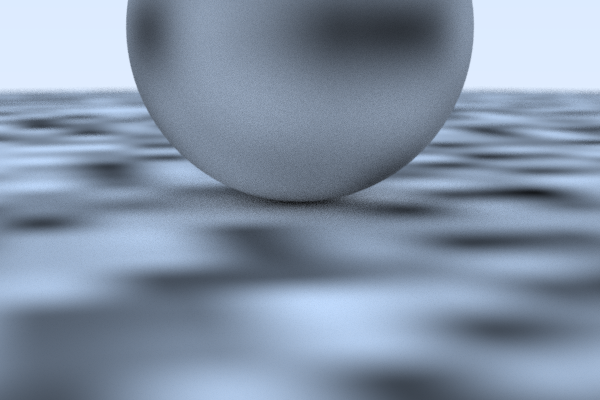and also: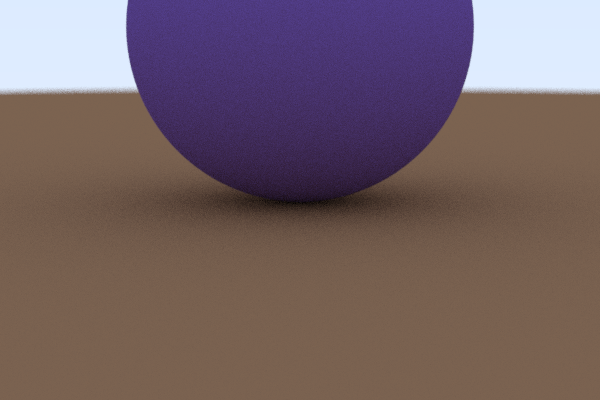In the problematic images at the beginning of the post, the lines are relatively straight (vertical) in parts of the image where the rows are generally relatively similar to each other (such as the bottom of the image), but the lines shift and bend more in more complex parts of the image, such as near the middle, where the small sphere is very close to the large "ground" sphere.

My understanding is that because the same random number generators were passed in at the start of rendering every row, the same "noise" was generated for each row. In rows with more complex interactions between the different objects (e.g., randomized diffuse reflections) in those rows cause the ray tracer to consume more random values from the generator stream as it renders each pixel in the row from left to right, thereby causing some of the randomly generated "noise" to appear sooner (e.g., farther to the left side or "sooner" in the row). At least, I think that would explain the particularly weird swirl effect near the point of contact at the origin.

Anyway, this was a fun bug to understand and to fix, and reminded me to be careful with random number generation.